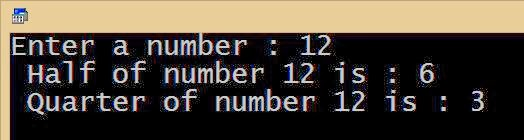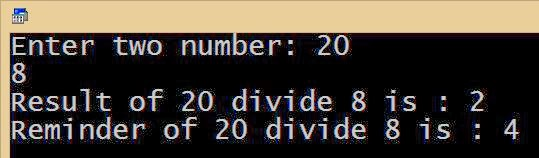# Arithmetic Operators in C

Arithmetic Operators:

Arithmetic operators are the common mathematical operator for mathematical operations. They are:

2.  – (Subtraction)
3.  * (Multiplication)
4.  / (Division)
5.  % (Modulus)

We already used this operator for adding two numbers. That is, this operator is using for adding two operands. This operator is mostly using for adding numeric values, also using for adding strings in some cases.

▶ Example: Program to accept four numbers and display their sum:

 #include #include void main() { int n1,n2,n3,n4,sum; clrscr(); printf(“Enter four numbers”); scanf(“%d %d %d %d”,&n1,&n2,&n3,&n4); sum = n1+n2+n3+n4; printf(“Sum of given four numbers is %d :”, sum); getch(); }

Explanation:

sum = n1+n2+n3+n4;

– Here sum of value of n1,n2,n3 and n4 added using plus operator then assigns result in to the variable ‘sum’.

Sample Output:Minus Operator (- for subtraction):

We already used this operator for subtracting two numbers. Here this operator subtracts given values. This operator is commonly using for subtracting numeric values.

▶ Example: Program to accept two numbers and find their sum. Then accept another number and subtract it from sum.

 #include #include void main() { int n1, n2, sum, num3, total; clrscr(); printf(“Enter two numbers”); scanf(“%d %d”,&n1,&n2); sum = n1+n2; printf(“Enter another number”); scanf(“%d”,&num3); total = sum – num3; printf(“Final result is %d :”, total); getch(); }

Code Explanation:

scanf(“%d %d “,&n1,&n2);

– Accepts first two numbers.

sum = n1+n2;

– Adds entered two numbers using plus operator and assigns sum in to the variable ‘sum’.

scanf(“%d”,&num3);

– Accepts another number in to the variable ‘num3’.

total = sum – num3;

– Here the third number num3 subtracts from above sum of first two numbers using minus operator and assigns the result in to the variable ‘total’.

Sample Output:Multiplication Operator (*):

We are familiar with this operator for multiplying two numbers. That is, this operator multiplies given values. This operator is commonly using for multiplying numeric values.

▶ Example: Program to accept a number, then find its double, triple, square and cube.

 #include #include void main() { int n, doubl, triple, square, cube; clrscr(); printf(“Enter a number :”); scanf(“%d”,&n); doubl = 2 * n; triple =3 * n; square = n * n; cube = n * n * n; printf(“n Given number is %d:”, n); printf(“n Its double is : %d”, doubl); printf(“n Its double is :%d”, triple); printf(“n Its double is :%d”, square); printf(“n Its double is :%d”, cube); getch(); }

Code Explanation:

scanf(“%d “,&n);

-Accepting given number and assigned to the variable ‘n’.

double = 2 * n;

– Double of a number is equal to multiplying a number with 2 . Here given number was multiplied with 2 and assigned result in to the variable ‘double’.

triple = 3 * n;

– Triple of a number is equal to multiplying a number with 3 (times). Here given number was multiplied with 3 and assigned result in to the variable ‘triple’.

square = n * n;

– Square of a number is multiplying a number with same number. Here entered number n was multiplied with same number n and assigned result into the variable ‘square’

cube = n * n * n;

– Cube of a number is multiplying a number with same number for 2 times. Here entered number n was multiplied with same number n for two time and assigned the result into the variable ‘cube’

Sample Output:Division Operator (/):

We are also familiar with this operator in division operation. This operator is commonly using for dividing numeric values.

▶ Example: Program to accept a number and find its half and quarter

 #include #include void main() { int num,half,quart; printf(“Enter a number :”); scanf(“%d”,&num); half = num / 2; quart = num / 4; printf(“Half of number %d is : %d “,num, half); printf(“n Quarter of number %d is : %d “,num, quart); getch(); }

Code Explanation:

half = num / 2;

– Half of a number is the dividing a number by 2. Here given number ‘num’ divides by 2 and assigns result into the variable ‘half’.

quart = num / 4;

– Quarter of a number is the dividing a number by 4. Here entered number ‘num’ divides by 4 and assigns result into the variable ‘quart’.

Sample Output:Modulus Operator (%):

This operator is not for finding percentage. This operator is using for finding reminder in a division operation. That is,

▶ 8 divides 2 ( 8 / 2), what is the answer ? Answer 4.

▶ If we divides 9 by 2 ( 9 / 2), what is the answer ? Answer is 4, but 1 is the reminder.

How do we get reminder?

9 / 2 = 4

4 x 2 = 8, then how many numbers need to reach to 9 ? Only 1 (9 – 8 = 1). That is, 1 is the reminder of this operation.

▶ 14 / 3 -> what is the reminder?

To find reminder we have to find its answer. In operation (14 / 3) we get answer 4.

4 x 3 = 12. Then how many numbers need to reach from answer (12) to 14? Only 2 (14 – 12 = 2). So here 2 is the reminder.

▶ 29 / 6 -> what is the reminder?

To find reminder we have to find the answer. In operation (29 / 6) we get answer 4.

4 x 6 = 24. Then how many numbers need to reach from answer (24) to 29? Only 5 (29 – 24 = 5). Here 5 is the reminder.

▶ In C, the symbol or operator % is used to find reminder of a mathematical operation.

That is the result of 9%2 is 1

Result of 14%3 is 2

Result of 29%6 is 4

Result of 34%7 is 6 and so on.

▶ Example: Program to accept two numbers and divide first number by second one, and find their division reminder.

 #include #include void main() { int num1,num2, result, remind; clrscr(); printf(“Enter two number:”); scanf(“%d%d”,&num1,&num2); result = num1 / num2; remind = num1% num2; printf(“Result of %d divide %d is : %d “,num1,num2,result); printf(“nReminder of %d divide %d is : %d “,num1,num2,remind); getch(); }

Code Explanation:

result = num1 / num2;

– Here num1 divides by num2 and result stores into the variable ‘result’.

remind = num1 % num2;

– Here reminder of num2 divides by num2 stores into the variable ‘remind’.

Sample Output: